Next: Characteristic polynomial Up: Regularity of matrix with Previous: Mathematical background   Contents

Implementation

A regularity test based on this approach is implemented as:

int ALIAS_Check_Regularity_Linear_Matrix(int DimA,
INTERVAL_VECTOR (* Func)(int l1, int l2,INTERVAL_VECTOR & v_IS),
int (* A_Cond)(int dimA,
INTERVAL_VECTOR (* Func1)(int l1, int l2,INTERVAL_VECTOR &v_IS),
INTERVAL_VECTOR & v_IS,INTERVAL_MATRIX &A),
int Row_Or_Column,
int Context,
INTEGER_MATRIX &Implication_Var,
int Use_Rohn,
INTERVAL_VECTOR &Domain)
where
• Row_Or_Column: 1 if the row of the matrix are used, 2 if the columns are used
• Context: is used to determine if this procedure is used according to the following rules (see section 7.4 for the meaning of the flag Simp_in_Cond):
• always used if 100 or if Context is equal to Simp_in_Cond
• not used if Context lie in [-2,2]
• not used if Context=3 and Simp_in_Cond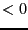• not used if Context=4 and Simp_in_Cond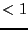• not used if Context=5 and Simp_in_Cond is not 0 or 1
• not used if Context=6 and Simp_in_Cond is not 0 or 2
• Implication_Var: an integer matrix of dimension DimA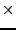, whereis the number of unknowns. If this matrix has a 1 at row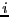, column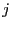, then the unknown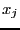appear linearly in some elements of row(or column) of the matrix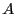• Use_Rohn: 1 if the Rohn consistency test is used to check that a matrix in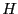has a constant sign
• Domain: the ranges for the input parameters
This procedure return -1 if all elements ofare regular, 0 otherwiseNext: Characteristic polynomial Up: Regularity of matrix with Previous: Mathematical background   Contents
Jean-Pierre Merlet 2012-12-20